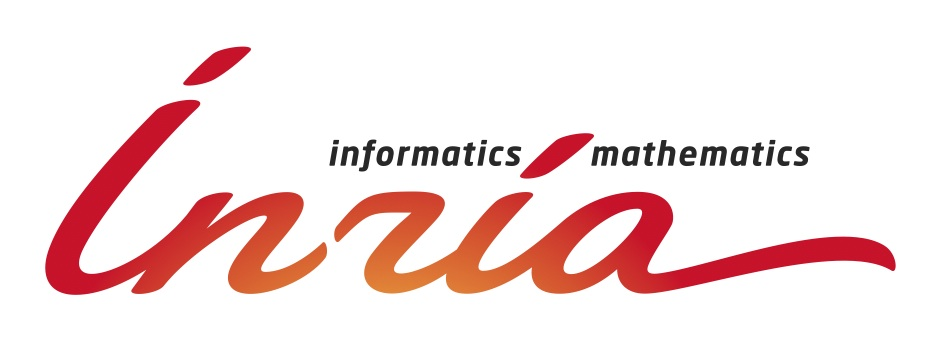International Spring School on

FORMALIZATION OF MATHEMATICS

(MAP 2012)

March, 12-16, 2012, Sophia Antipolis,France## Overview and topics

A growing population of mathematicians, computer scientists, and engineers use computers to construct and verify proofs of mathematical results. Among the various approaches to this activity, a fruitful one relies on interactive theorem proving. When following this approach, researchers have to use the formal language of a theorem prover to encode their mathematical knowledge and the proofs they want to scrutinize. The mathematical knowledge often contains two parts: a static part describing structures and a dynamic part describing algorithms. Then proofs are made in a style that is inspired from usual mathematical practice but often differs enough that it requires some training. A key ingredient for the mathematical practitioner is the amount of mathematical knowledge that is already available in the system's library.

The Coq system is an interactive theorem prover based on Type Theory. It was recently used to study the proofs of advanced mathematical results. In particular, it was used to provide a mechanically verified proof of the four-colour theorem and it is now being used in a long term effort, called Mathematical Components to verify results in group theory, with a specific focus on the odd order theorem, also known as the Feit-Thompson theorem. These two examples rely on a structured library that covers various aspects of finite set theory, group theory, arithmetic, and algebra.

The aim of this school is to give mathematicians and mathematically inclined researchers the keys to the Coq system and the Mathematical Components library. The topics covered are:

• Formal proof techniques
• Structuration of libraries
• Encoding of common mathematical structures
• Formal description of algorithms
• An overview of advanced projects:
• The odd order theorem
• Constructive algebraic topology
• Kepler's conjecture,
• Differential calculus,
• Foundational investigations.

The school's contents will be organized as a balanced schedule between lectures and laboratory sessions where participants will be invited to work on their own computer and try their hands on a progressive collection of exercises.

## Speakers

The current list of speakers is:

• Georges Gonthier (Microsoft Research)
• Thomas C. Hales (University of Pittsburgh)
• Julio Rubio (Universidad de La Rioja)
• Bas Spitters (Radboud Universiteit, Nijmegen)